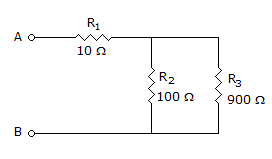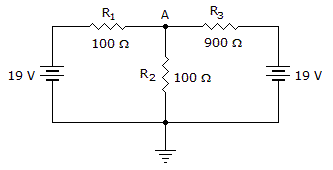# Electronics - Series-Parallel Circuits

### Exercise :: Series-Parallel Circuits - Filling the Blanks

11.In the given circuit, the total resistance (RT) between points A and B equals _____.

 A. 10B. 100C. 900D. 1,010Explanation:

No answer description available for this question. Let us discuss.

12.In the given circuit, the voltage at point A equals ______.

 A. –10 V B. +1 V C. +9 V D. +10 V

Explanation:

No answer description available for this question. Let us discuss.

13.If the voltage between points A and B equals 9 V in the given circuit, then VR3 equals ______.

 A. 0.9 V B. 8.1 V C. 9 V D. More information is needed to find VR3.